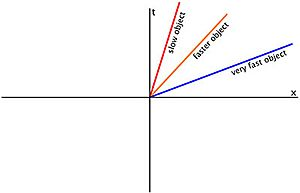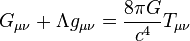# World line facts for kids

Kids Encyclopedia FactsThe distinct paths of three objects going at different speeds and their respective measurements of the passage of time, where the t axis represents the passage of time, and the x axis represents the speed of the object.

A world line is the unique path that an object has as it travels through both space and time, usually called spacetime. As we learn from special relativity, the faster an object goes, the more time slows down for that object. As you can see in the illustration to the right, the slower object has a quicker passage of time than the very fast object, the one which time passes much more slowly for. When an object reaches the speed of light, it will be zero on the t axis, meaning that it will have made no progress in the time direction. Basically, the world lines show that when the speed of light is reached, time stops for the observer. World lines are very often used in theoretical physics and special relativity, as well as general relativity.

## Usage

The concept of world lines is used widely in theoretical physics, for it shows some interesting facts about high-speed motion. For example, the time dilation equation presented by Albert Einstein is algebraically undefined when an object's velocity is the speed of light, but using world lines one can find that when the speed is the speed of light, time will stop. Although Einstein's equation (for time dilation) does show that an object going faster than light goes backward in time, the same concept can be described using world lines.

General relativity$G_{\mu \nu} + \Lambda g_{\mu \nu}= {8\pi G\over c^4} T_{\mu \nu}$
Einstein field equations

## Images for kidsWorld line Facts for Kids. Kiddle Encyclopedia.